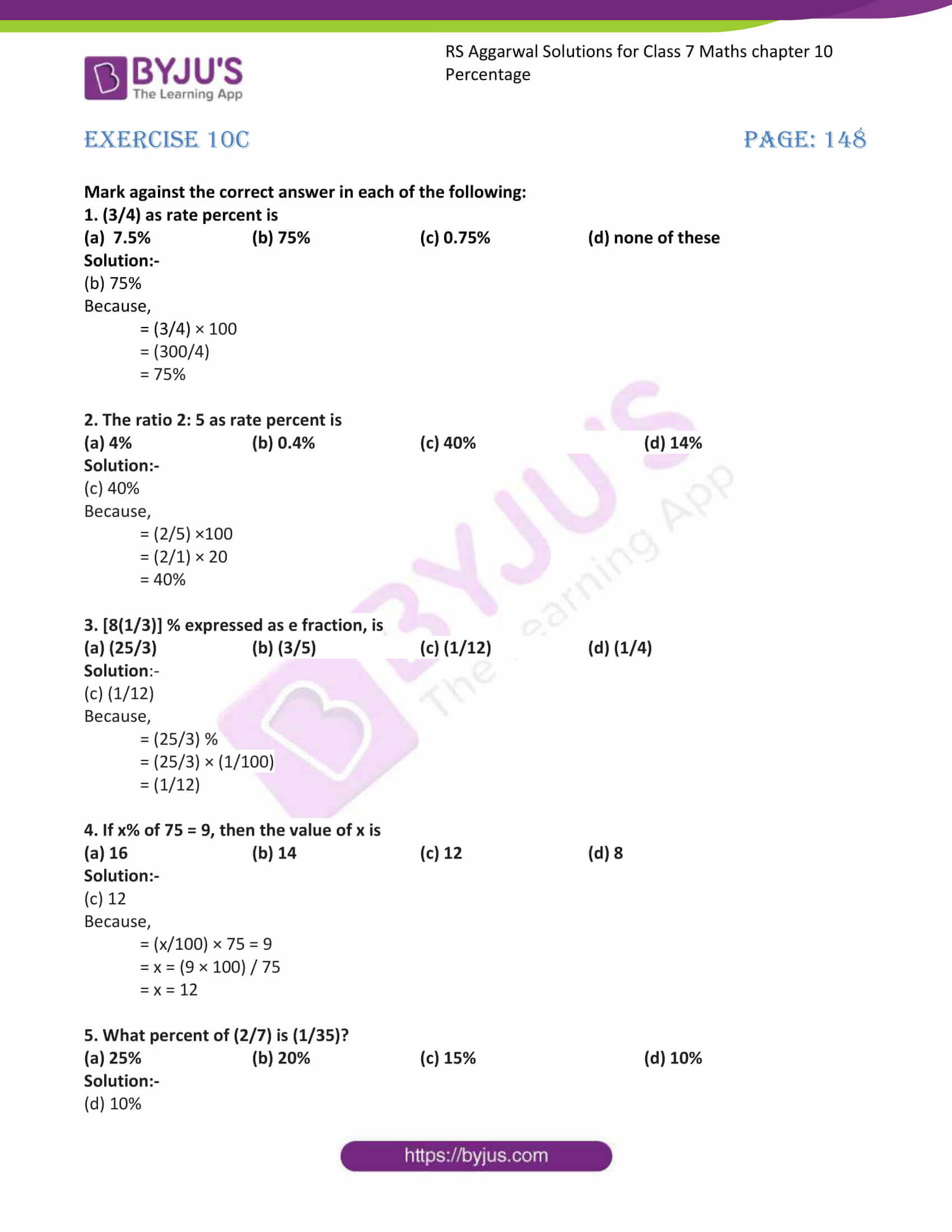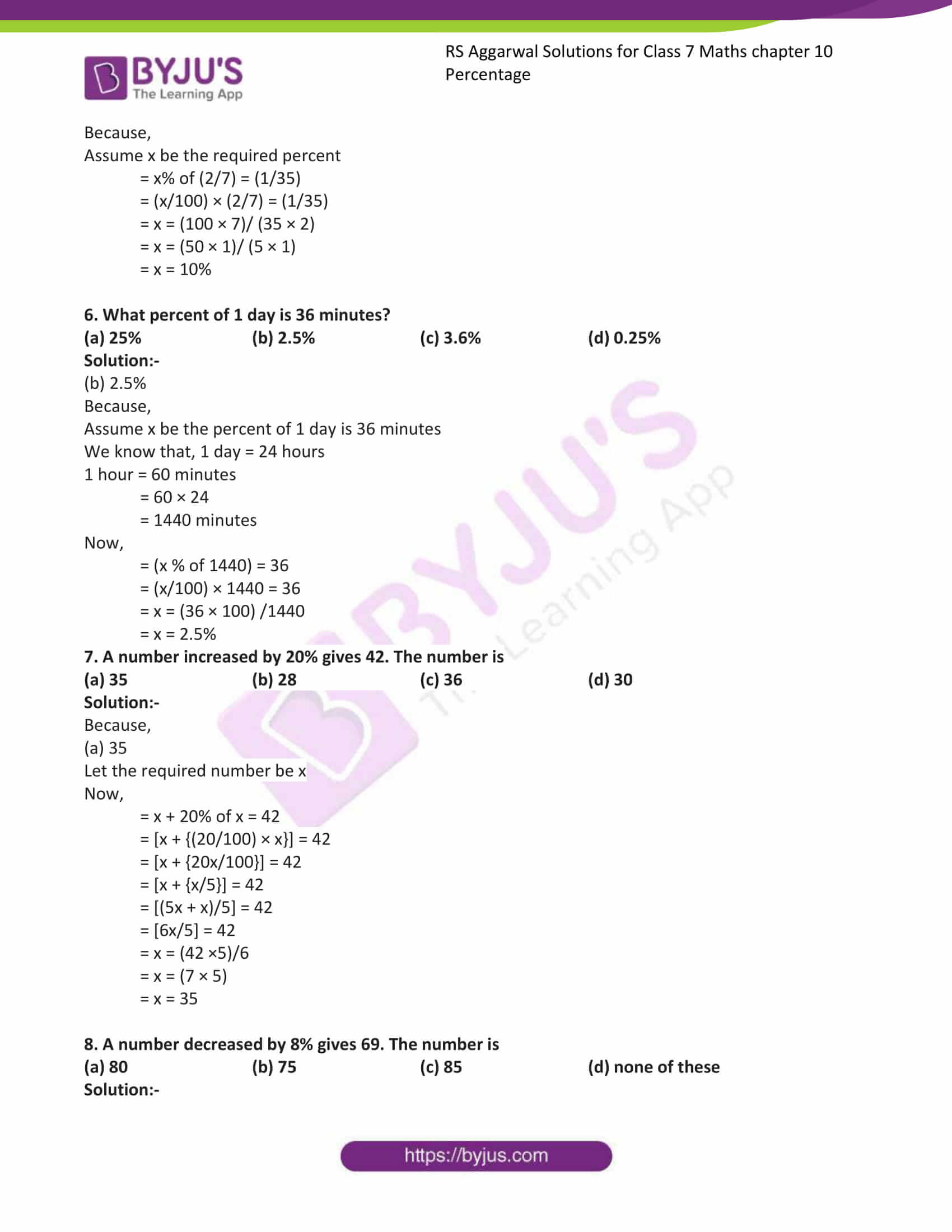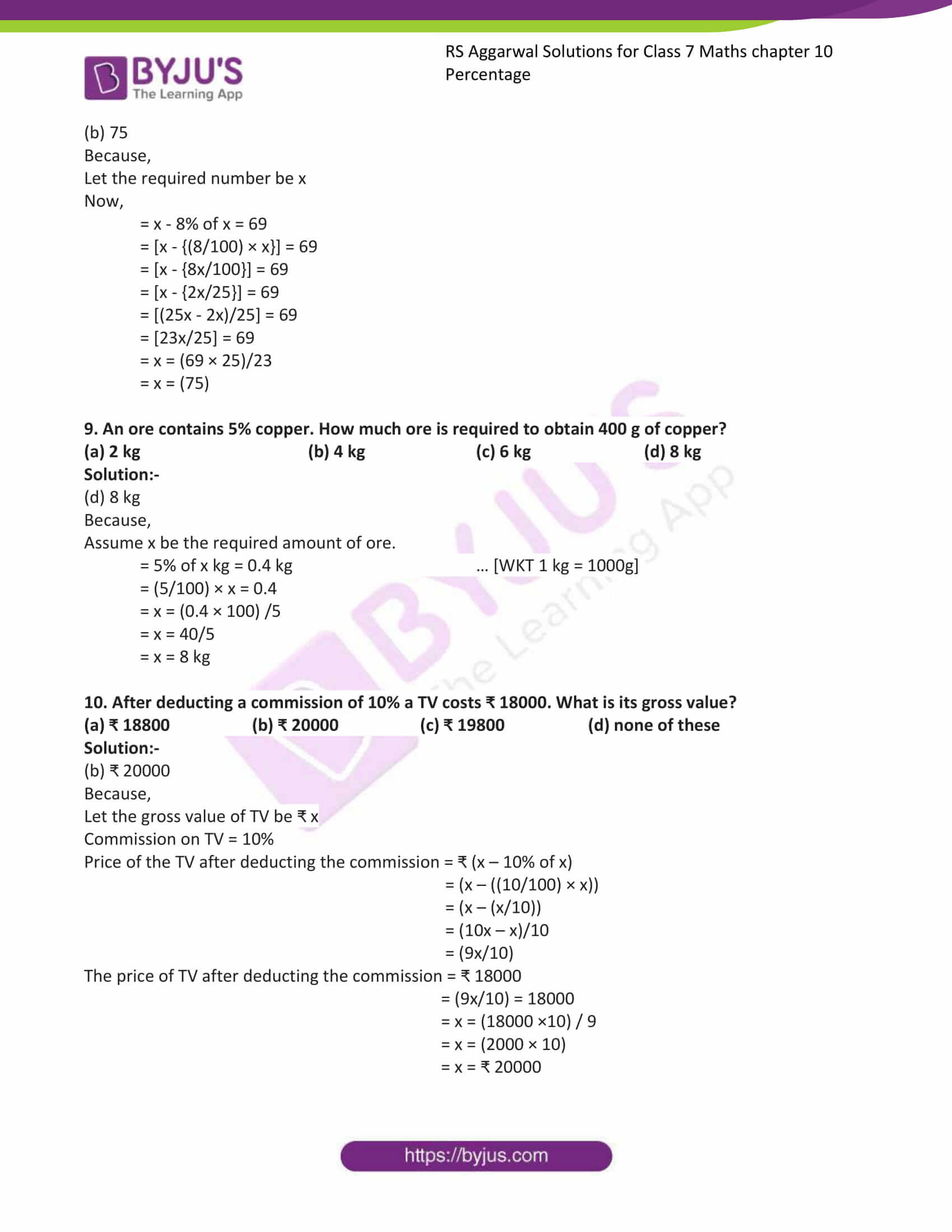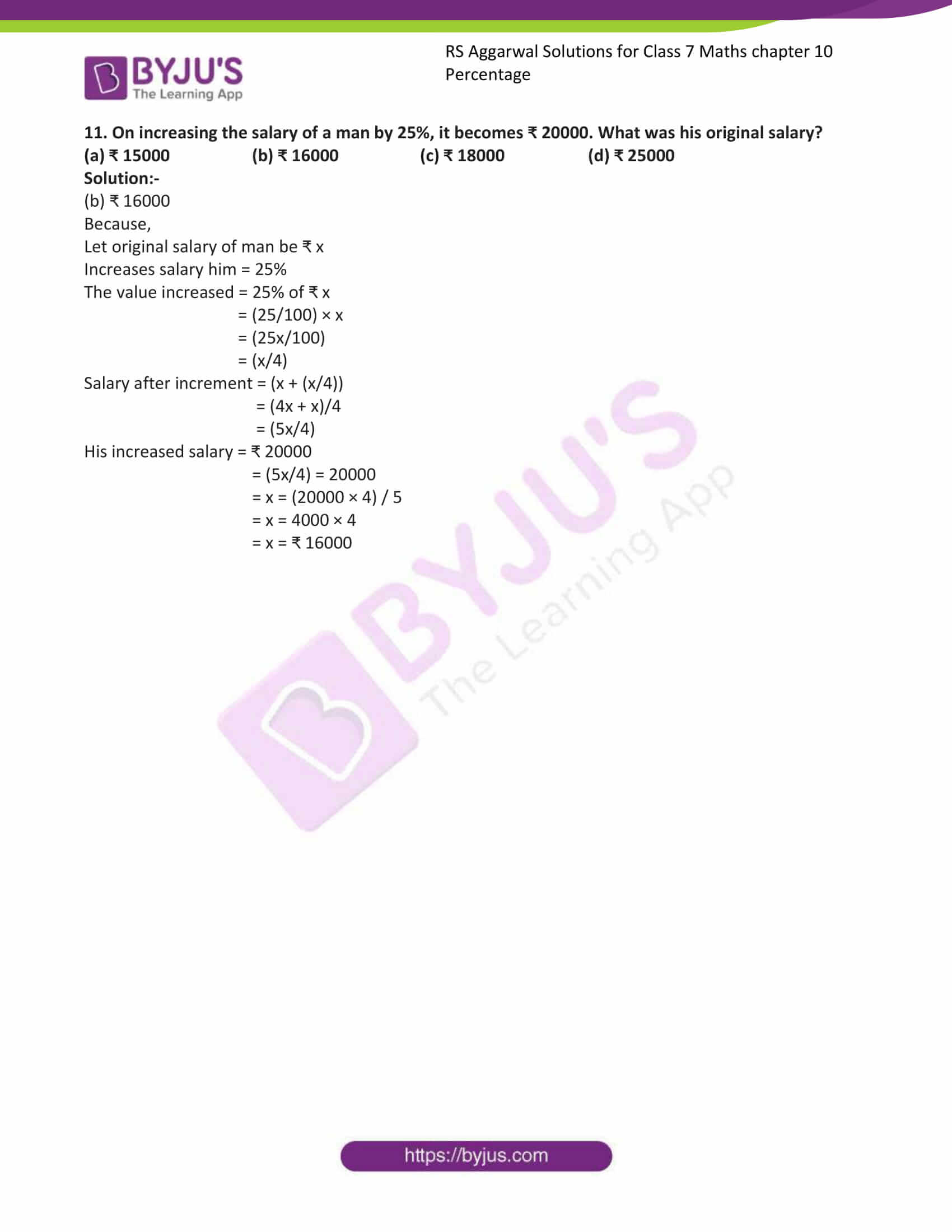# RS Aggarwal Solutions for Class 7 Maths Exercise 10C Chapter 10 Percentage

RS Aggarwal Solutions for Exercise 10C of Class 7 Maths Chapter 10 Percentage are available here. This exercise is entirely of objective type questions. It includes all topics present in the RS Aggarwal Solutions for Class 7 Maths Chapter 10 Percentage. After solving these objective type questions, students can absorb more knowledge from the chapter and consequently, score high-grades in Maths.

## Download the PDF of RS Aggarwal Solutions For Class 7 Maths Chapter 10 Percentage – Exercise 10C### Access answers to Maths RS Aggarwal Solutions for Class 7 Chapter 10 – Percentage Exercise 10C

Mark against the correct answer in each of the following:

1. (3/4) as rate percent is

(a) 7.5% (b) 75% (c) 0.75% (d) none of these

Solution:-

(b) 75%

Because,

= (3/4) × 100

= (300/4)

= 75%

2. The ratio 2: 5 as rate percent is

(a) 4% (b) 0.4% (c) 40% (d) 14%

Solution:-

(c) 40%

Because,

= (2/5) ×100

= (2/1) × 20

= 40%

3. [8(1/3)] % expressed as e fraction, is

(a) (25/3) (b) (3/5) (c) (1/12) (d) (1/4)

Solution:-

(c) (1/12)

Because,

= (25/3) %

= (25/3) × (1/100)

= (1/12)

4. If x% of 75 = 9, then the value of x is

(a) 16 (b) 14 (c) 12 (d) 8

Solution:-

(c) 12

Because,

= (x/100) × 75 = 9

= x = (9 × 100) / 75

= x = 12

5. What percent of (2/7) is (1/35)?

(a) 25% (b) 20% (c) 15% (d) 10%

Solution:-

(d) 10%

Because,

Assume x be the required percent

= x% of (2/7) = (1/35)

= (x/100) × (2/7) = (1/35)

= x = (100 × 7)/ (35 × 2)

= x = (50 × 1)/ (5 × 1)

= x = 10%

6. What percent of 1 day is 36 minutes?

(a) 25% (b) 2.5% (c) 3.6% (d) 0.25%

Solution:-

(b) 2.5%

Because,

Assume x be the percent of 1 day is 36 minutes

We know that, 1 day = 24 hours

1 hour = 60 minutes

= 60 × 24

= 1440 minutes

Now,

= (x % of 1440) = 36

= (x/100) × 1440 = 36

= x = (36 × 100) /1440

= x = 2.5%

7. A number increased by 20% gives 42. The number is

(a) 35 (b) 28 (c) 36 (d) 30

Solution:-

Because,

(a) 35

Let the required number be x

Now,

= x + 20% of x = 42

= [x + {(20/100) × x}] = 42

= [x + {20x/100}] = 42

= [x + {x/5}] = 42

= [(5x + x)/5] = 42

= [6x/5] = 42

= x = (42 ×5)/6

= x = (7 × 5)

= x = 35

8. A number decreased by 8% gives 69. The number is

(a) 80 (b) 75 (c) 85 (d) none of these

Solution:-

(b) 75

Because,

Let the required number be x

Now,

= x – 8% of x = 69

= [x – {(8/100) × x}] = 69

= [x – {8x/100}] = 69

= [x – {2x/25}] = 69

= [(25x – 2x)/25] = 69

= [23x/25] = 69

= x = (69 × 25)/23

= x = (75)

9. An ore contains 5% copper. How much ore is required to obtain 400 g of copper?

(a) 2 kg (b) 4 kg (c) 6 kg (d) 8 kg

Solution:-

(d) 8 kg

Because,

Assume x be the required amount of ore.

= 5% of x kg = 0.4 kg … [WKT 1 kg = 1000g]

= (5/100) × x = 0.4

= x = (0.4 × 100) /5

= x = 40/5

= x = 8 kg

10. After deducting a commission of 10% a TV costs ₹ 18000. What is its gross value?

(a) ₹ 18800 (b) ₹ 20000 (c) ₹ 19800 (d) none of these

Solution:-

(b) ₹ 20000

Because,

Let the gross value of TV be ₹ x

Commission on TV = 10%

Price of the TV after deducting the commission = ₹ (x – 10% of x)

= (x – ((10/100) × x))

= (x – (x/10))

= (10x – x)/10

= (9x/10)

The price of TV after deducting the commission = ₹ 18000

= (9x/10) = 18000

= x = (18000 ×10) / 9

= x = (2000 × 10)

= x = ₹ 20000

11. On increasing the salary of a man by 25%, it becomes ₹ 20000. What was his original salary?

(a) ₹ 15000 (b) ₹ 16000 (c) ₹ 18000 (d) ₹ 25000

Solution:-

(b) ₹ 16000

Because,

Let original salary of man be ₹ x

Increases salary him = 25%

The value increased = 25% of ₹ x

= (25/100) × x

= (25x/100)

= (x/4)

Salary after increment = (x + (x/4))

= (4x + x)/4

= (5x/4)

His increased salary = ₹ 20000

= (5x/4) = 20000

= x = (20000 × 4) / 5

= x = 4000 × 4

= x = ₹ 16000

### Access other exercises of RS Aggarwal Solutions For Class 7 Chapter 10 – Percentage

Exercise 10A Solutions

Exercise 10B Solutions